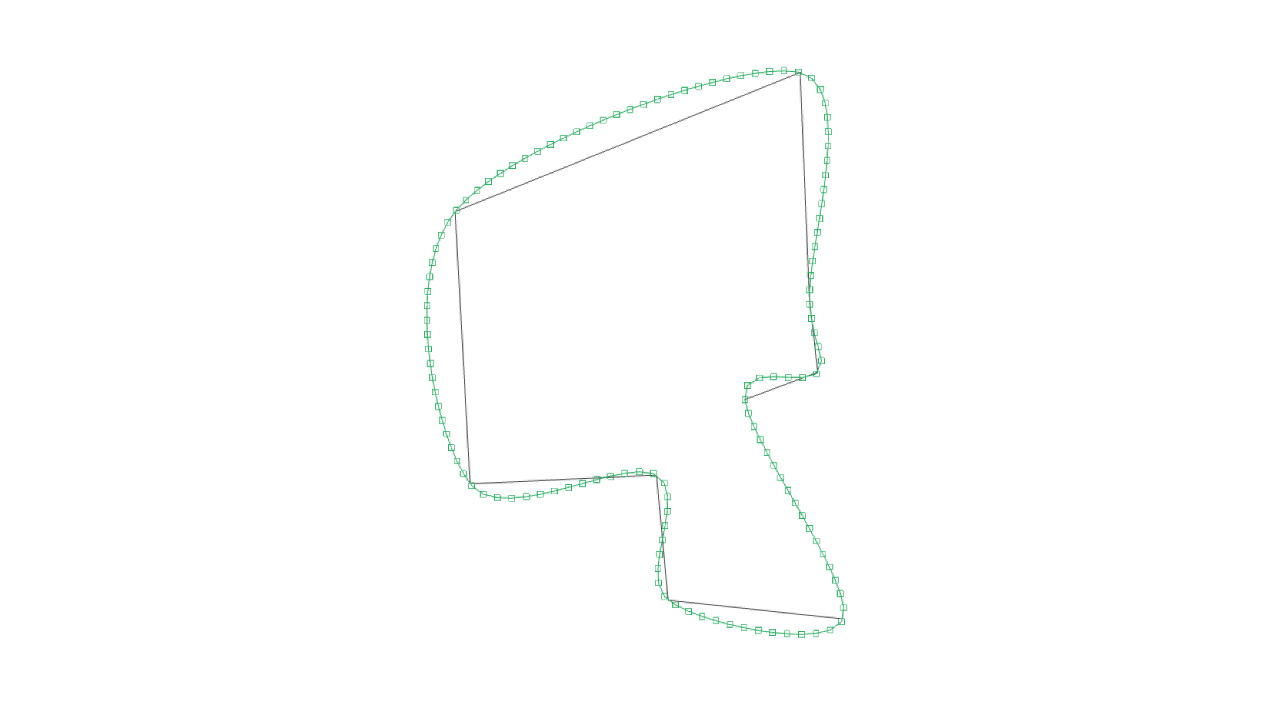# spline-points

Subdivides a 3d path into spline points

# Example

``````npm install spline-points --save
``````
``````const splinePoints = require('spline-points')

const points = [[0, 0, 0], [1, 0, 0], [2, 1, 0]]
const smoothPoints = splinePoints(points, { segmentLength: 0.1 })
// smoothPoints = [[0, 0, 0], [0.09, -0.01, 0], [0.19, -0.02, 0], ...]
``````

### Running the example

``````cd spline-points/example
npm install
budo index.js --open
``````

# API

``````var splinePoints = require('spline-points')
``````

### `smoothPoints = splinePoints(points, opts)`

Subdivides a 3d path into spline points

• `points` : Array of vec3 - path of 3d points [x, y, z] to subdivide
• `opts`
• `closed` : Bool - is the path closed?, `false`
• `segmentLength` : Number - distance between generated points